## STUDY ON INSTABILITY OF THE WIDE VANELESS DIFFUSER WITH DIFFERENT TREATMENTS OF VISCOSITY 1)

School of Mechanical Engineering,Beijing Institute of Technology,Beijing 100081, China

 基金资助: 1) 国家自然科学基金资助项目.  51736001

Received: 2019-07-29   Accepted: 2019-09-18   Online: 2019-09-18Abstract

The global stability of vaneless diffuser in the centrifugal compressor is often influenced by the main flow stability, backflow at exit and the boundary layer separation. For the vaneless diffuser with large axial width ratio, the effect of the main flow and boundary layer on the instability perturbations is the main subject. In this paper, the mean flow of the wide vaneless diffuser is firstly obtained with numerical simulations. The Eulers' and Navier-Stokes equations are linearized respectively based on the small perturbations assumption. Then the inviscid stability approach considering the inviscid main flow and the mixed stability approach considering the effect of both eddy viscosity and molecular viscosity are established. The prediction results are validated against the experimental results. At last, the structural sensitivity based on the adjoint method is adopted. And the wave-maker region is revealed under different treatments of viscosity. The instability perturbations are located at the middle of the flow field when inviscid main flow is only considered. And a centrifugal instability maybe the main cause of the vaneless diffuser stall. When the inviscid main flow and boundary layer are both considered, the wave-maker region not only lies at the main flow near the middle of the vaneless diffuser, but also lies at the reverse flow region of the boundary layer.

Keywords： wide vaneless diffuser ; flow instability ; eigenvalue ; sensitivity analysis

Hu Chenxing, Yang Ce. STUDY ON INSTABILITY OF THE WIDE VANELESS DIFFUSER WITH DIFFERENT TREATMENTS OF VISCOSITY 1). Chinese Journal of Theoretical and Applied Mechanics[J], 2019, 51(6): 1775-1784 DOI:10.6052/0459-1879-19-207

## 引言

### 图1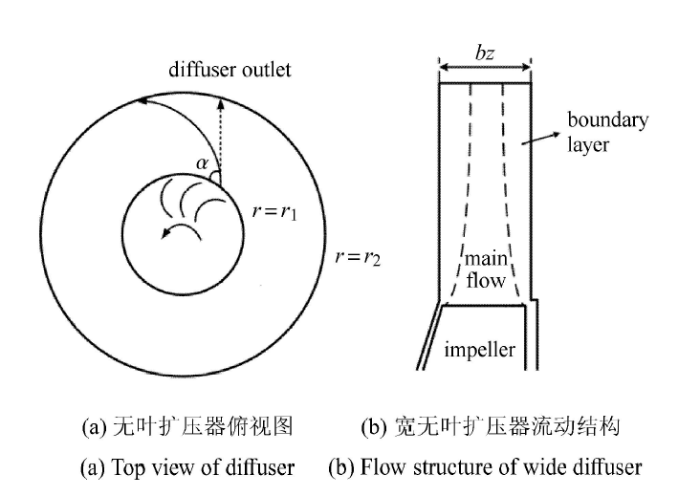Fig.1   Sketch of the flow in the vaneless diffuser

Jansen、Senoo 等[17-18]、Frigne 等认为无叶扩压器内的不稳定性主要是由壁面边界层分离现象诱发的.Senoo 与 Kinoshita通过对三维边界层进行计算,考虑了两侧壁面边界层混合和入口的轴向非对称性影响,建立了经验公式,确定了回流与失速产生时的入口流动角度的关系.对于宽无叶扩压器内三维湍流边界层的作用,Tsurusaki 与 Munakata通过建立边界层厚度预测模型,结合实验测量的方法,在宽扩压器失稳发生前捕捉到壁面回流区的局部速度扰动,如图2 所示.他们认为该现象证明了边界层回流对于无叶扩压器失稳的关键作用.

### 图2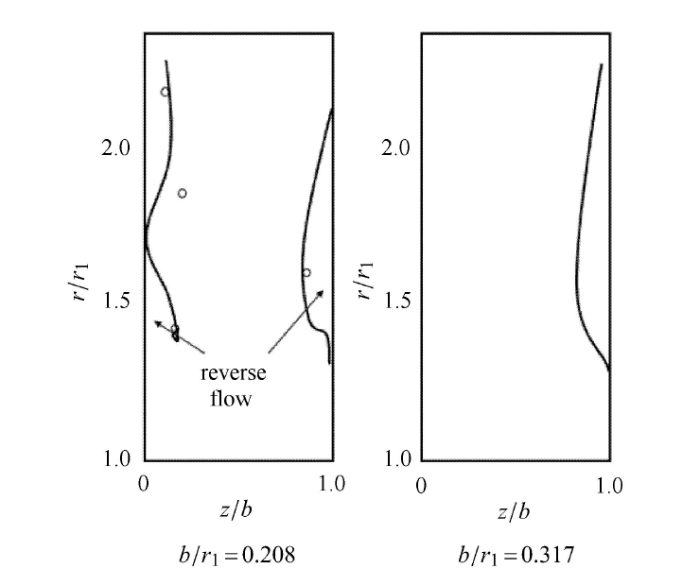Fig.2   The reverse flow regions by prediction and experiment in Tsurusaki and Munakata's work

Dou 与 Mizuki 基于 Johnson的边界层三角模型,提出了一种计算宽无叶扩压器内三维湍流边界层的方法.该方法假设扩压器流道内径向速度的分布为二阶梯形,并将动量方程与主流关系式联立,并预测得到了不同入流关键角度下边界层分离点的径向位置,如图3 所示.他们将理论预测结果与实验测量进行对比分析,认为当壁面径向回流发展至无叶扩压器入口,并与射流-尾流区域相互作用时,才会产生无叶扩压器内的旋转失速.但是应该注意到,在孤立的无叶扩压器的实验或数值计算中,也会有失稳的产生 . 窦华书  针对不同宽度比 的无叶扩压器失稳机理进行了解释,他认为对于较宽的无叶扩压器,扩压器不会容易导致级的喘振,叶轮大概率会优先于扩压器发生失速;对于较小宽度扩压器,失速既可能先发生于叶轮,又可能先发生于无叶扩压器,此时限制扩压器稳定性的是流动损失.

### 图3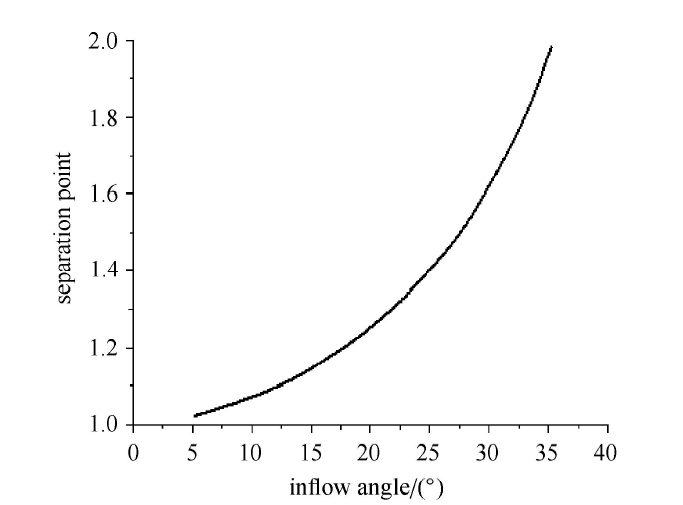Fig.3   The separation point position predicted by Dou

## 1 理论方法

### 图4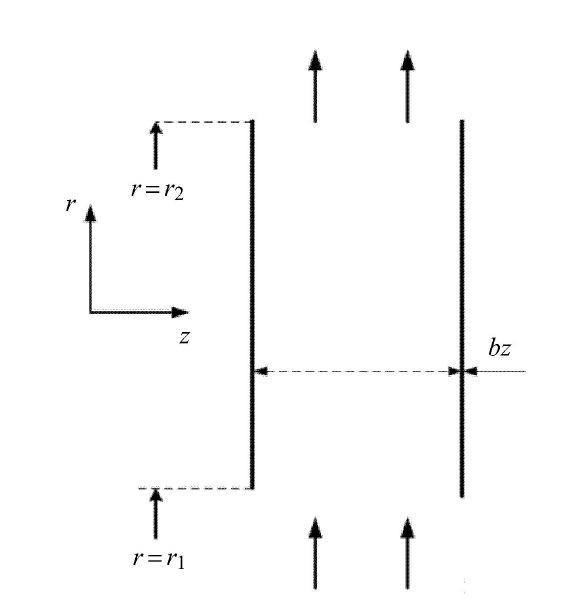Fig.4   The sketch of the vaneless diffuser model

### 1.2 方程的线性化

$\frac{\partial {\pmb u}}{\partial t} + {\pmb u} \cdot \nabla {\pmb u} = - \nabla p +\frac{1}{Re}\nabla ^2{\pmb u}$
$\nabla \cdot {\pmb u} = 0$

Table 1  Non-dimensionalization of the parameters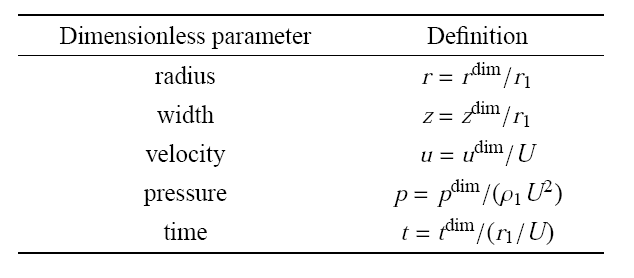${\pmb u} (x,t) = \bar{\pmb u} (x) + \tilde{\pmb u} (x,t) +{\pmb u}'(x,t)$

$\bar{\pmb u} \cdot \nabla \bar{\pmb u} = - \nabla \bar {p} + \frac{1}{Re}\nabla^2\bar{\pmb u} - \nabla \cdot (\underbrace {\overline {{\pmb u}'{\pmb u}'} }_{\tau ^{\rm P}} + \underbrace {\overline {\tilde {u}\tilde {u}} }_{\tau ^{\rm C}})$
$\nabla \cdot \bar {\pmb u} = 0$

$\frac{\partial \tilde {\pmb u}}{\partial t} + \tilde {\pmb u} \cdot \nabla \bar {\pmb u} + \bar {\pmb u} \cdot \nabla \tilde {\pmb u} = \\ \qquad - \nabla \tilde {p} + \frac{1}{Re}\nabla ^2\tilde {\pmb u} + \nabla \cdot(\underbrace {\overline {\tilde {\pmb u}\tilde {\pmb u}} - \tilde {\pmb u}\tilde {\pmb u}}_{\tau^N}) - \nabla \cdot (\underbrace {\left\langle {{\pmb u}'{\pmb u}'} \right\rangle - \overline {{\pmb u}'{\pmb u}'} }_\tau )$

$\tau = - 2\mu _{\rm t} (\nabla + \nabla ^{\rm T})\tilde {\pmb u}$

$\frac{\partial \tilde {\pmb u}}{\partial t} + \tilde {\pmb u} \cdot \nabla \bar {\pmb u} +\bar {\pmb u} \cdot \nabla \tilde {\pmb u} = - \nabla \tilde {p} + \nabla \cdot \left[ {\frac{1}{Re_{\rm eff} }(\nabla + \nabla ^T)\tilde {\pmb u}} \right]$
$\nabla \cdot \tilde {\pmb u} = 0$

(1) 扩压器入口与壁面

$\tilde {\pmb u} = 0$

(2) 扩压器出口

$\tilde {p} = 0$

$\tilde {\pmb u} = \hat {\pmb u}(r,z){\rm e}^{{\rm i}( - \omega t + m\theta )}$
$\tilde {p} = \hat {p}(r,z) {\rm e}^{{\rm i} ( - \omega t + m\theta )}$

$- \omega \hat {\pmb u} + L\hat {\pmb u} + \nabla \hat {p} = 0$
$\nabla \cdot \hat {\pmb u} = 0$
$L\hat {\pmb u} = \hat {\pmb u} \cdot \nabla \bar {\pmb u} + \bar {\pmb u} \cdot \nabla \hat{\pmb u} - \nabla \cdot \left[ {\frac{1}{Re_{\rm eff } }(\nabla + \nabla ^{\rm T})\hat {\pmb u}} \right]$

${\pmb M}\hat {\pmb q} = \omega{\pmb J}\hat{\pmb q}$

### 1.3 伴随方程与结构敏感性

$\left\langle { {\pmb l}, {\pmb n}} \right\rangle = {\pmb l}^{\rm T}{\pmb O}{\pmb n }= \int_\varOmega {\pmb l}^{\rm T}{\pmb n} d\varOmega$

$\left\langle {\pmb l}, {\pmb A}{\pmb n} \right\rangle = {\pmb l}^{\rm T}{\pmb O}{\pmb A}{\pmb O}^{ - 1}{\pmb O}{\pmb n} = \\\ \qquad ({\pmb O}^{ - 1}{\pmb A}^{\rm T}{\pmb O}{\pmb l})^{\rm T}{\pmb O}{\pmb n} = \left\langle{\pmb O}^{ - 1}{\pmb A}^{\rm T}{\pmb O}{\pmb l}, {\pmb n}\right\rangle = \left\langle {\pmb A}^\dagger {\pmb l}, {\pmb n} \right\rangle$

$\left\langle{\pmb l}, {\pmb A}{\pmb n} \right\rangle = \left\langle {\pmb A}^\dagger {\pmb l}, {\pmb n} \right\rangle$

$\omega ^\dagger \hat {\pmb u}^\dagger + L^\dagger \hat {\pmb u}^\dagger + \nabla \hat {p}^\dagger = 0$
$\nabla \cdot \hat {\pmb u}^\dagger = 0$
$L^\dagger \hat {\pmb u}^\dagger = \hat {\pmb u}^\dagger \cdot (\nabla \bar {\pmb u})^{\rm T} - \bar {\pmb u} \cdot \nabla \hat {\pmb u}^\dagger - \nabla \cdot [\frac{1}{Re_{\rm eff} }(\nabla + \nabla ^{\rm T})\hat {\pmb u}^\dagger ]$

${\pmb E}\hat{\pmb q}^\dagger = \omega ^\dagger {\pmb F}\hat {\pmb q}^\dagger$

${\pmb S} = \left\| \hat {\pmb u} \right\| \cdot \left\| {\hat {\pmb u}^\dagger } \right\|$

## 2 平均流动

### 2.1 无黏平均流动

$\bar {v}_r / r + \partial \bar {v}_r / \partial r = 0$
$\bar {v}_r \cdot \partial \bar {v}_r / \partial r - \bar {v}_\theta ^2 / r = - \partial \bar {p} / \partial r$
$\bar {v}_r \cdot \partial \bar {v}_\theta / \partial r + \bar {v}_r\bar {v}_\theta / r = 0$
$\bar {v}_r \cdot \partial \bar {v}_z / \partial r = 0$

### 图5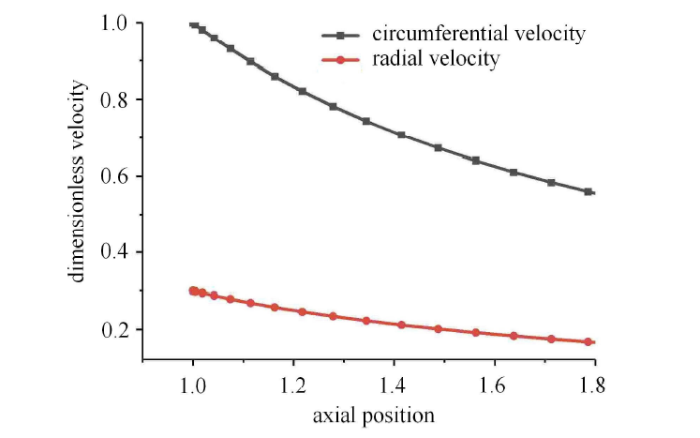Fig.5   The inviscid mean flow of the vaneless diffuser

### 图6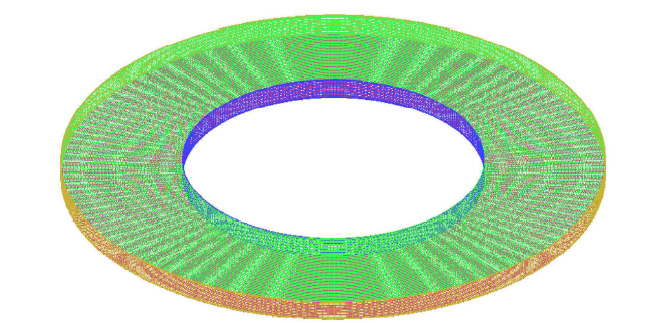Fig.6   Mesh of the isolated vaneless diffuser

### 图7Fig.7   The turbulent mean flow of the vaneless diffuser

Table 2  Parameters for the numerical simulations of mean flow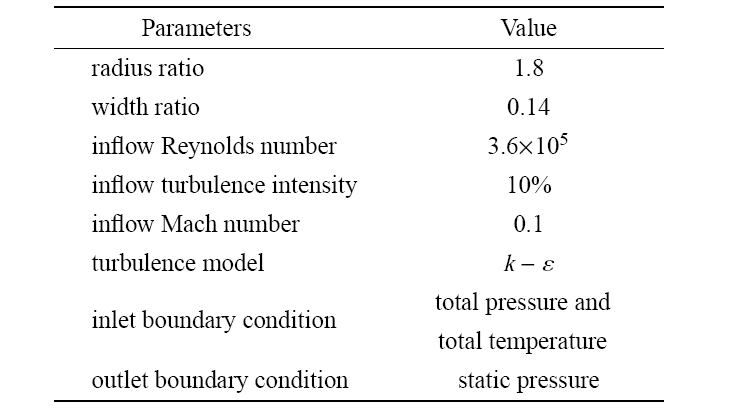### 图8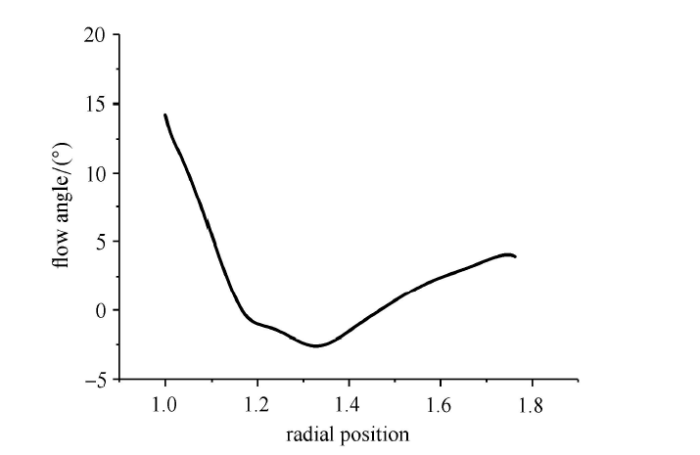Fig.8   The flow angle at 99 % width of the vaneless diffuser

## 3 稳定性分析

### 图9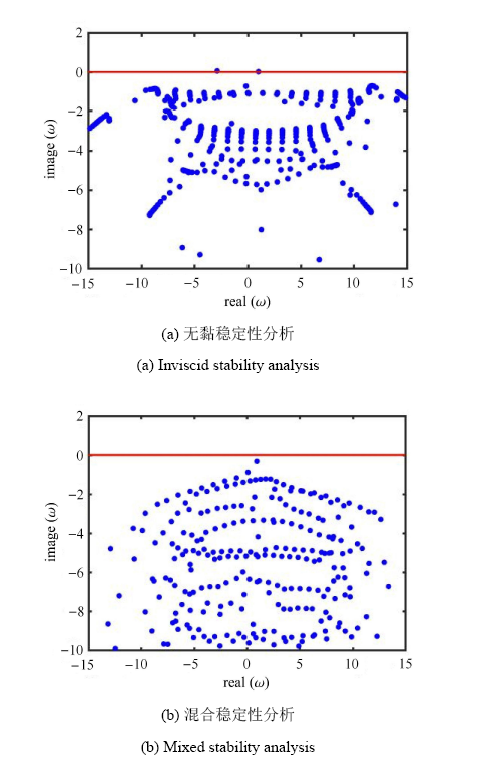Fig.9   The eigenvalue spectrum calculated with inviscid and mixed stability

Table 3  Prediction validation against the experimental results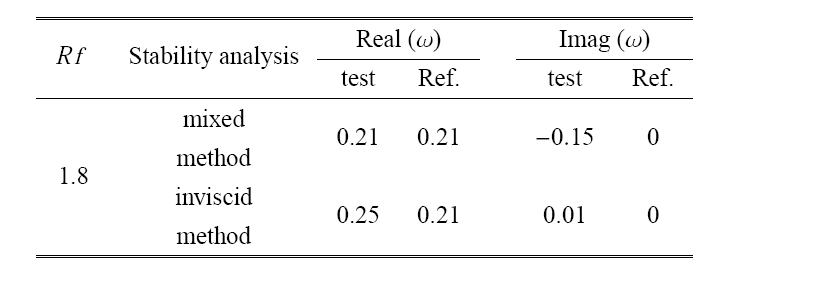### 图10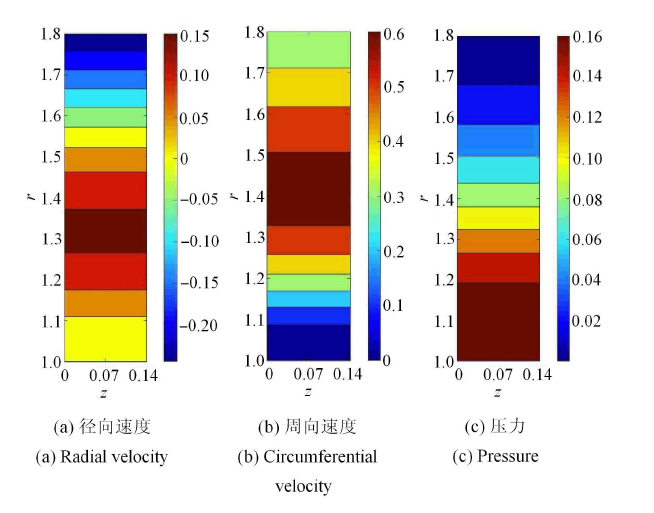Fig.10   The eigenmodes of the inviscid stability analysis

### 图11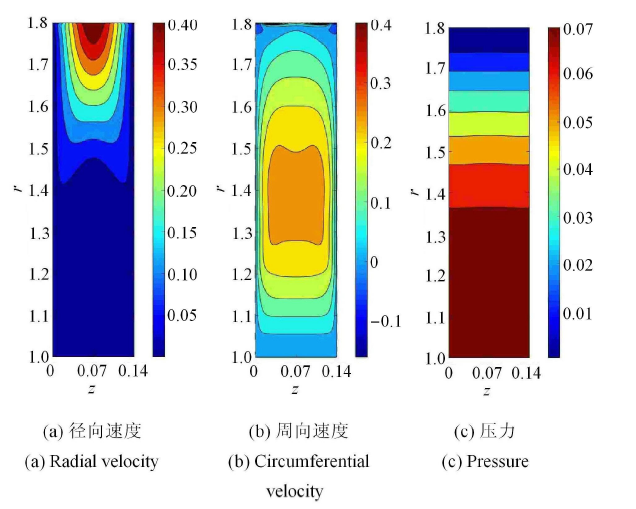Fig.11   The eigenmodes of the mixed stability analysis

## 4 伴随模态与结构敏感性

### 图12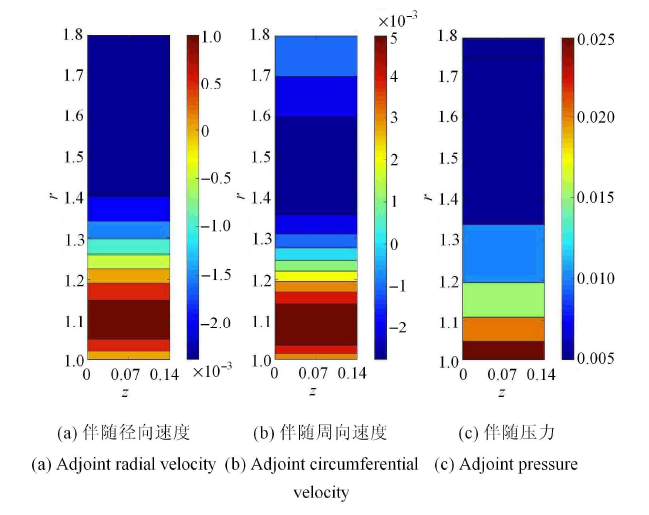Fig.12   The adjoint eigenmodes of the inviscid analysis

### 图13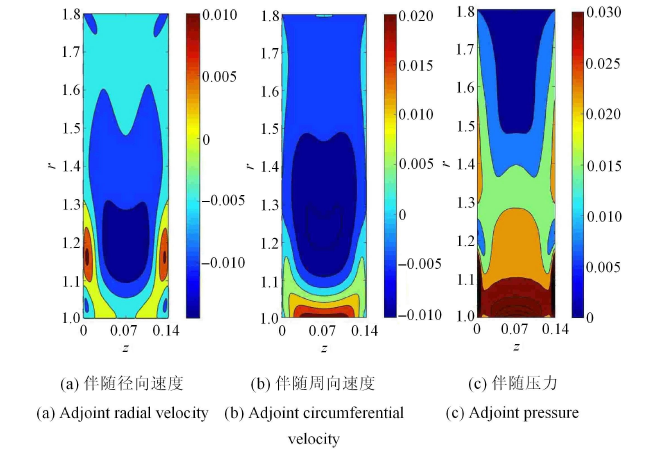Fig.13   The adjoint eigenmodes of the mixed stability analysis

### 图14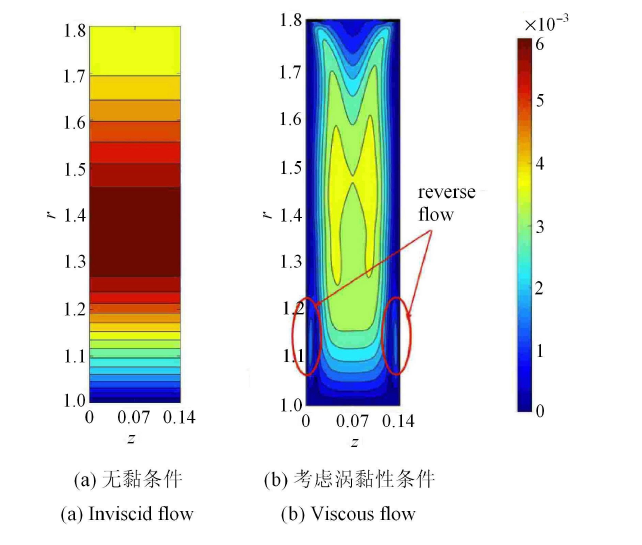Fig.14   The structural sensitivity of the vaneless diffuser flow

## 5 结论与总结

(1)本文在考虑涡黏性条件下建立的混合稳定性分析方法,相比无黏稳定性分析方法,可以更为为准确的预测得到宽无叶扩压器失稳扰动的旋转速度,而采用两种方法预测所得的失速团个数一致.

(2)在无黏条件下,径向半径比 1.8,宽度比 0.14 的宽扩压器失稳来源于径向位置 $r=1.3\sim 1.5$ 的流动区域,为一种二维离心失稳;在考虑黏性作用时,宽无叶扩压器的全局不稳定扰动在核心主流与径向位置 $r=1.1 \sim 1.2$ 处壁面径向回流的共同作用下诱发.

(3)不稳定扰动的诱发与放大,不仅与当地扰动的强弱有关,而且与该空间位置流动对扰动的感受性相关.

## 参考文献 原文顺序 文献年度倒序 文中引用次数倒序 被引期刊影响因子

( Zhu Baozhen, Guo Tao. Centrifugal Compressor Xi' an: Xi'an Jiaotong University Press Xi'an Jiaotong University Press, 1989 (in Chinese))

[博士论文]. 上海: 上海交通大学, 2011

( Gao Chuang .

Investigation and analysis of vaneless diffuser stall and system surge precursor. [PhD Thesis]

Shanghai: Shanghai Jiao Tong University, 2011 (in Chinese))

Schumann L F.

A Three-dimensional axisymmetric calculation procedure for turbulent flows in a radial vaneless diffuser

Journal of Engineering for Gas Turbines & Power, 1984,108(1):118-124

URL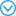To reveal the effect of high-temperature creep on the blade-tip radial running clearance of aeroengine high-pressure turbines, a distributed collaborative generalized regression extremum neural network is proposed by absorbing the heuristic thoughts of distributed collaborative response surface method and the generalized extremum neural network, in order to improve the reliability analysis of blade-tip clearance with creep behavior in terms of modeling precision and simulation efficiency. In this method, the generalized extremum neural network was used to handle the transients by simplifying the response process as one extremum and to address the strong nonlinearity by means of its nonlinear mapping ability. The distributed collaborative response surface method was applied to handle multi-object multi-discipline analysis, by decomposing one &quot;big&quot; model with hyperparameters and high nonlinearity into a series of &quot;small&quot; sub-models with few parameters and low nonlinearity. Based on the developed method, the blade-tip clearance reliability analysis of an aeroengine high-pressure turbine was performed subject to the creep behaviors of structural materials, by considering the randomness of influencing parameters such as gas temperature, rotational speed, material parameters, convective heat transfer coefficient, and so forth. It was found that the reliability degree of the clearance is 0.9909 when the allowable value is 2.2 mm, and the creep deformation of the clearance presents a normal distribution with a mean of 1.9829 mm and a standard deviation of 0.07539 mm. Based on a comparison of the methods, it is demonstrated that the proposed method requires a computing time of 1.201 s and has a computational accuracy of 99.929% over 104 simulations, which are improvements of 70.5% and 1.23%, respectively, relative to the distributed collaborative response surface method. Meanwhile, the high efficiency and high precision of the presented approach become more obvious with the increasing simulations. The efforts of this study provide a promising approach to improve the dynamic reliability analysis of complex structures.

Rodgers C, Mnew H.

Experiments with a model free rotating vaneless diffuser

Journal of Engineering for Gas Turbines & Power, 1975,97(2):231-245

URLTo reveal the effect of high-temperature creep on the blade-tip radial running clearance of aeroengine high-pressure turbines, a distributed collaborative generalized regression extremum neural network is proposed by absorbing the heuristic thoughts of distributed collaborative response surface method and the generalized extremum neural network, in order to improve the reliability analysis of blade-tip clearance with creep behavior in terms of modeling precision and simulation efficiency. In this method, the generalized extremum neural network was used to handle the transients by simplifying the response process as one extremum and to address the strong nonlinearity by means of its nonlinear mapping ability. The distributed collaborative response surface method was applied to handle multi-object multi-discipline analysis, by decomposing one &quot;big&quot; model with hyperparameters and high nonlinearity into a series of &quot;small&quot; sub-models with few parameters and low nonlinearity. Based on the developed method, the blade-tip clearance reliability analysis of an aeroengine high-pressure turbine was performed subject to the creep behaviors of structural materials, by considering the randomness of influencing parameters such as gas temperature, rotational speed, material parameters, convective heat transfer coefficient, and so forth. It was found that the reliability degree of the clearance is 0.9909 when the allowable value is 2.2 mm, and the creep deformation of the clearance presents a normal distribution with a mean of 1.9829 mm and a standard deviation of 0.07539 mm. Based on a comparison of the methods, it is demonstrated that the proposed method requires a computing time of 1.201 s and has a computational accuracy of 99.929% over 104 simulations, which are improvements of 70.5% and 1.23%, respectively, relative to the distributed collaborative response surface method. Meanwhile, the high efficiency and high precision of the presented approach become more obvious with the increasing simulations. The efforts of this study provide a promising approach to improve the dynamic reliability analysis of complex structures.

Yang C, Zhang D, MA C.

Investigation into the interaction of centrifugal compressor impeller and vaneless diffuser

Journal of Beijing Institute of Technology (English Edition), 2006,15(3):273-277

( Ma Chao, Wang Hang, Liu Yungang,

Numerical simulation and flow analysis of the vaneless diffuser of centrifugal compressor in low flow condition

I.C.E.& Powerplant, 2010, ( 5):14-16 (in Chinese))

( Guo Qiang, Zhu Xiaocheng, Du Zhaohui,

Three-dimensional numerical simulation for rotating stall inside high-speed centrifugal compressor

Journal of Propulsion Technology, 2007,28(4):373-377 (in Chinese))

( Gao Jun, Li Jia,

Numerical investigation of mode exchange in hypersonic boundary layer

Chinese Journal of Theoretical and Applied Mechanics, 2018,50(6):1368-1378 (in Chinese))

( Tong Fulin, Li Xin, Yu Changping, et al.

Direct numerical simulation of hypersonic shock wave and turbulent boundary layer interactions

Chinese Journal of Theoretical and Applied Mechanics, 2018,50(2):197-208 (in Chinese))

( Cui Guangyao, Pan Chong, Gao Qi, et al.

Flow structure in the turbulent boundary layer over directional riblets surfaces

Chinese Journal of Theoretical and Applied Mechanics, 2017,49(6):1201-1212 (in Chinese))

Moore FK.

Weak rotating flow disturbances in a centrifugal compressor with a vaneless diffuser

Journal of Turbomachinery, 1989,111:442-449

Shen F, Zhu XC, Liu PY, et al.

A three-dimensional compressible flow model on vaneless diffuser stall

Journal of Shanghai Jiaotong University, 2011,45(11):1725-1730

URLA three-dimensional compressible flow model was presented to study the occurrence of rotating waves in vaneless diffusers of centrifugal compressors. Solutions to the discrete 3D Euler equations for compressible flow were obtained via singular value decomposition (SVD) method. Critical inlet mass flow rate of vaneless diffuser stall and relative rotating speed of stall cell were calculated. The effects of inlet Mach number were studied and the results show that both critical inlet mass flow rate and rotating wave speed vary with inlet Mach number especially when the diffuser is long. In addition, critical inlet mass flow rate and rotating wave speed are also influenced by diffuser outlet/inlet radius ratio, departure from axial uniform distribution of the undisturbed radial velocity at the diffuser inlet, and impeller back swept angle. Finally, a comparison with experimental results in open literature was made, and the results of 3D model show better agreement than those of 2D model.

Tsujimoto Y, Yoshida Y, Mori Y.

Study of vaneless diffuser rotating stall based on two-dimensional inviscid flow analysis

Journal of Fluids Engineering, 1996,118(1):123-127

Abdelhamid AN.

Analysis of rotating stall in vaneless diffusers of centrifugal compressors

Canadian Aeronautics and Space Journal, 1980,26:118-128

Ljevar S .

Rotating stall in wide vaneless diffusers

[Master Thesis]. Ann Arbor: Technische Universiteit Eindhoven (The Netherlands), 2007

Jansen W.

Rotating stall in a radial vaneless diffuser

ASME Journal of Basic Engineering, 1964,86(4):750-758

Senoo Y, Kinoshita Y.

Influence of inlet flow condition and geometries of a centrifugal vaneless diffuser on critical flow angle for reverse flow

Journal of Fluids Engineering, 1977,99(1):102-103

Senoo Y, Kinoshita Y .

Limits of rotating stall and stall in vaneless diffuser of centrifugal compressors//ASME 1978 Gas Turbine Conference and Products Show, London,

England, 1978

Frigne P, Braembussche RVD.

A theoretical model for rotating stall in the vaneless diffuser of a centrifugal compressor

Journal of Engineer for Gas Turbines & Power, 1985,107(2):507-513

URLTo reveal the effect of high-temperature creep on the blade-tip radial running clearance of aeroengine high-pressure turbines, a distributed collaborative generalized regression extremum neural network is proposed by absorbing the heuristic thoughts of distributed collaborative response surface method and the generalized extremum neural network, in order to improve the reliability analysis of blade-tip clearance with creep behavior in terms of modeling precision and simulation efficiency. In this method, the generalized extremum neural network was used to handle the transients by simplifying the response process as one extremum and to address the strong nonlinearity by means of its nonlinear mapping ability. The distributed collaborative response surface method was applied to handle multi-object multi-discipline analysis, by decomposing one &quot;big&quot; model with hyperparameters and high nonlinearity into a series of &quot;small&quot; sub-models with few parameters and low nonlinearity. Based on the developed method, the blade-tip clearance reliability analysis of an aeroengine high-pressure turbine was performed subject to the creep behaviors of structural materials, by considering the randomness of influencing parameters such as gas temperature, rotational speed, material parameters, convective heat transfer coefficient, and so forth. It was found that the reliability degree of the clearance is 0.9909 when the allowable value is 2.2 mm, and the creep deformation of the clearance presents a normal distribution with a mean of 1.9829 mm and a standard deviation of 0.07539 mm. Based on a comparison of the methods, it is demonstrated that the proposed method requires a computing time of 1.201 s and has a computational accuracy of 99.929% over 104 simulations, which are improvements of 70.5% and 1.23%, respectively, relative to the distributed collaborative response surface method. Meanwhile, the high efficiency and high precision of the presented approach become more obvious with the increasing simulations. The efforts of this study provide a promising approach to improve the dynamic reliability analysis of complex structures.

Tsurusaki H, Munakata A.

A study on the rotating stall in vaneless diffusers of centrifugal fans. 2nd report, an analysis of pressure fluctuations

Nihon Kikai Gakkai Ronbunshu B Hen/transactions of the Japan Society of Mechanical Engineers Part B, 1987,53(487):885-893

Dou H, Mizuki S.

Analysis of the flow in vaneless diffusers with large width-to-radius ratios

Journal of Turbomachinery, 1998,120(1):193-201

Johnston JP.

On the three-dimensional turbulent boundary layer generated by secondary flow

Journal of Fluids Engineering, 1960,82(1):233-246

URL&nbsp;提出了一种离心压缩机系统内无叶扩压器失速的三维理论模型.该模型采用线性化的三维欧拉方程分析扩压器内的流体不可压缩流动，采用有限差分法和奇异值分裂(SVD)法进行求解，以预测无叶扩压器失速的临界来流角和失速团相对转速.结果表明，在三维情况下，无叶扩压器失速的临界来流角和失速团相对转速除了受到扩压器几何尺寸的影响以外，还受到扩压器入口流体平均径向速度的轴向分布的影响.与二维模型相比，三维模型所得到的预测结果与实验结果更相符.

( Shen Feng, Zhu Xiaocheng, Du Zhaohui.

A three-dimensional stall model for vaneless diffusers of centrifugal compression system

Journal of Shanghai Jiaotong University, 2011(9):1251-1255 (in Chinese))

URL&nbsp;提出了一种离心压缩机系统内无叶扩压器失速的三维理论模型.该模型采用线性化的三维欧拉方程分析扩压器内的流体不可压缩流动，采用有限差分法和奇异值分裂(SVD)法进行求解，以预测无叶扩压器失速的临界来流角和失速团相对转速.结果表明，在三维情况下，无叶扩压器失速的临界来流角和失速团相对转速除了受到扩压器几何尺寸的影响以外，还受到扩压器入口流体平均径向速度的轴向分布的影响.与二维模型相比，三维模型所得到的预测结果与实验结果更相符.

( Dou Huashu,

The stability regimes of radial vaneless diffuser

Journal of Engineering Thermophysics, 1994,15(2):166-169 (in Chinese))

Gudmundsson K, Tim C.

Instability wave models for the near-field fluctuations of turbulent jets

Journal of Fluid Mechanics, 2011,689(689):97-128

Barkley D.

Linear analysis of the cylinder wake mean flow

Epl, 2006,75(5):194-205

Turton E, Tuckerman S, Barkley D.

Prediction of frequencies in thermosolutal convection from mean flows

Physical Review E Statistical Nonlinear & Soft Matter Physics, 2015,91(4):043009

URLWe study a dynamical system consisting of two mutually coupled molecular lasers, each of which shows mixed-mode oscillations and chaos when uncoupled. The type of coupling, incoherent laser interaction via saturable absorbers is an example of inhibitory nonlinear coupling, which is also found in Hodgkin-Huxley models that describe action potentials in neurons. We have carried out extensive numerical bifurcation analysis and numerical simulations to show that for small-enough coupling, well below the chaotic synchronization threshold, the presence of distinctive resonances in a symmetric mirror configuration of the system generates a type of rare events characterized by very small amplitudes. When this symmetry is broken by introducing a relatively small difference between the lasers pump parameters near an in-phase Hopf bifurcation, we observe extreme rare events (rogue waves) in one of the lasers. In this case the outliers deviate from power-law distributions and are reminiscent of those known as dragon kings. We consider the conditions for both types of rare events to occur, their origin, as well as relevant statistical features.

Giannetti F, Luchini P.

Structural sensitivity of the first instability of the cylinder wake

Journal of Fluid Mechanics, 2007,581(581):167-197

Fani A, Camarri S, Salvetti MV.

Stability analysis and control of the flow in a symmetric channel with a sudden expansion

Physics of Fluids, 2012,24(8):084102

Juniper P.

The effect of confinement on the stability of viscous planar jets and wakes

Journal of Fluid Mechanics, 2010,656(656):309-336

Rukes L, Paschereit O, Oberleithner K.

An assessment of turbulence models for linear hydrodynamic stability analysis of strongly swirling jets

European Journal of Mechanics - B/Fluids, 2016,59:205-218

Oberleithner K, Paschereit O, Wygnanski I.

On the impact of swirl on the growth of coherent structures

Journal of Fluid Mechanics, 2014,741(2):156-199

Turton E, Tuckerman S, Barkley D.

Prediction of frequencies in thermosolutal convection from mean flows

Physical Review E Statistical Nonlinear & Soft Matter Physics, 2015,91(4):043009

URLWe study a dynamical system consisting of two mutually coupled molecular lasers, each of which shows mixed-mode oscillations and chaos when uncoupled. The type of coupling, incoherent laser interaction via saturable absorbers is an example of inhibitory nonlinear coupling, which is also found in Hodgkin-Huxley models that describe action potentials in neurons. We have carried out extensive numerical bifurcation analysis and numerical simulations to show that for small-enough coupling, well below the chaotic synchronization threshold, the presence of distinctive resonances in a symmetric mirror configuration of the system generates a type of rare events characterized by very small amplitudes. When this symmetry is broken by introducing a relatively small difference between the lasers pump parameters near an in-phase Hopf bifurcation, we observe extreme rare events (rogue waves) in one of the lasers. In this case the outliers deviate from power-law distributions and are reminiscent of those known as dragon kings. We consider the conditions for both types of rare events to occur, their origin, as well as relevant statistical features.

Tammisola O, Juniper P .

Adjoint sensitivity analysis of hydrodynamic stability in a gas turbine fuel injector//ASME Turbo Expo 2015: Turbine Technical Conference and Exposition, Montréal,

 〈〉